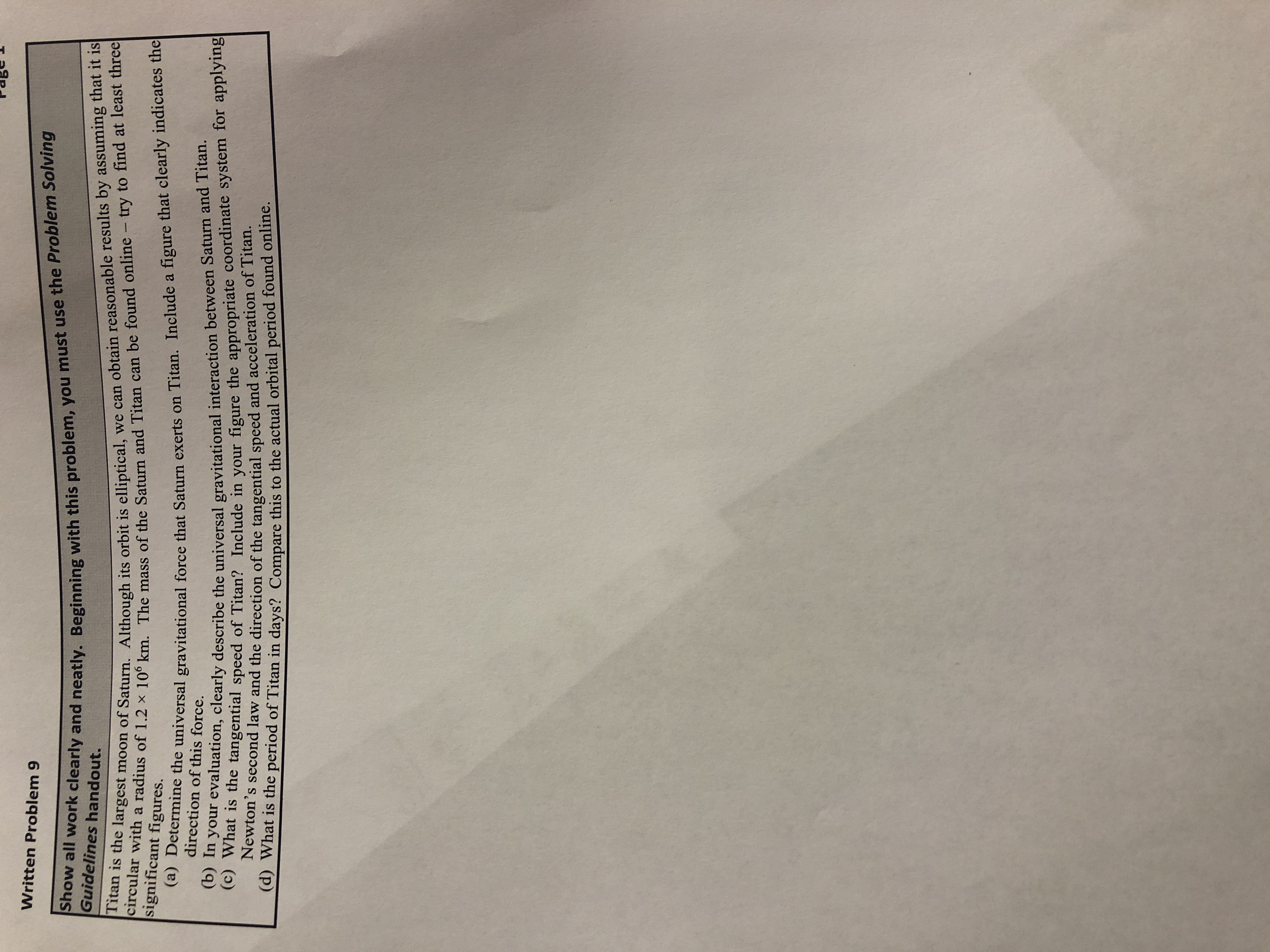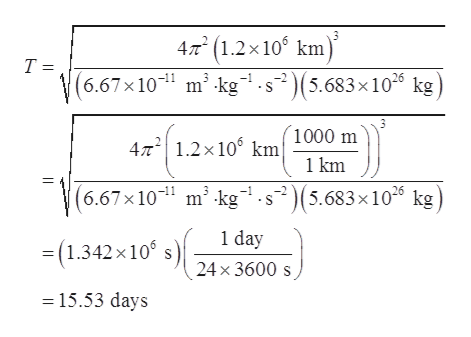# Written Problem 9Show all work clearly and neatly. Beginning with this problem, you must use the Problem SolvingGuidelines handout.Titan is the largest moon of Saturn. Although its orbit is elliptical, we can obtain reasonable results by assuming that it iscircular with a radius of 1.2 x 10° km. The mass of the Saturn and Titan can be found online - try to find at least threesignificant figures.(a) Determine the universal gravitational force that Saturn exerts on Titan. Include a figure that clearly indicates thedirection of this force.(b) In your evaluation, clearly describe the universal gravitational interaction between Saturn and Titan.(c) What is the tangential speed of Titan? Include in your figure the appropriate coordinate system for applyingNewton's second law and the direction of the tangential speed and acceleration of Titan.(d) What is the period of Titan in days? Compare this to the actual orbital period found online.

Question

PART Dhelp_outlineImage TranscriptioncloseWritten Problem 9 Show all work clearly and neatly. Beginning with this problem, you must use the Problem Solving Guidelines handout. Titan is the largest moon of Saturn. Although its orbit is elliptical, we can obtain reasonable results by assuming that it is circular with a radius of 1.2 x 10° km. The mass of the Saturn and Titan can be found online - try to find at least three significant figures. (a) Determine the universal gravitational force that Saturn exerts on Titan. Include a figure that clearly indicates the direction of this force. (b) In your evaluation, clearly describe the universal gravitational interaction between Saturn and Titan. (c) What is the tangential speed of Titan? Include in your figure the appropriate coordinate system for applying Newton's second law and the direction of the tangential speed and acceleration of Titan. (d) What is the period of Titan in days? Compare this to the actual orbital period found online. fullscreen
check_circle

Step 1

The formula for the orbital period is:

Step 2

Mass of the Saturn is 5.683×1026 kg.

Substitu...help_outlineImage Transcriptionclose4/m(1.2x10 km T = (6.67x10 m kg s2(5.683x10 kg 1000 m 4T2 1.2x106 km 1 km (6.67x10 m2 kg.s(5.683x10 kg 1 day (1.342x 10 24x 3600s 15.53 days fullscreen

### Want to see the full answer?

See Solution

#### Want to see this answer and more?

Solutions are written by subject experts who are available 24/7. Questions are typically answered within 1 hour.*

See Solution
*Response times may vary by subject and question.
Tagged in

### Rotational Mechanics